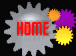Glossary

Catalyst – A substance present in small amounts relative to the reactants that increases the rate of a chemical reaction without being consumed in the process.

Channel – A tubular passage (groove) for fluid.

Concentration - The amount of a specified substance in a unit amount of another substance.

Conversion rate – The rate in which the reactants react to produce the products.

Endothermic – A chemical reaction which absorbs heat.

Exothermic - A chemical reaction which gives off heat.

FIDAP – A finite element modeling software package that can be used to solve fluid flow problems with either 2-D or 3-D geometries for a variety of situations (e.g., laminar vs. turbulent) and equations (e.g., momentum, energy) over time.

Finite difference method (FDM) – A method of modeling physical situations by breaking up one large problem into many smaller (and easier) problems. A grid of points (nodes) is placed on top of the geometry being modeled. The governing equations of the system under investigation are solved for each node at each time point in an iterative fashion until the final time point is reached. The governing equations can be very complicated when applied to the entire system as a whole, but can be written as a system of algebraic equations when applied to each node individually.

Finite element model (FEM) – Like the finite difference method, FEM is a method of modeling physical situations by breaking up one large problem into many smaller (and easier) problems. FEM is used in structural analysis and computational fluid mechanics. The geometry being examined is divided into small regions (2-D for 2-D models, 3-D for 3-D models). The governing equations of the system are solved for each element, rather than for the entire system all at once, which allows the user to simply the equations. This method is computationally intense and time-consuming.

Frictional force – The force between the fluid and the pipe walls, in which the pipe walls resist the motion of the fluid.

Heat capacity – The amount of heat required to raise the temperature of a body by 1 degree, either at constant pressure or constant volume and without inducing a chemical change or change of phase.

Heat of reaction (formation) – The amount of heat absorbed or given off during the formation of a given molecule. Usually given in terms of energy per mol.

Isobaric – System is maintained at a constant pressure.

Isothermal - system is maintained at a constant temperature.

Laminar – Low Reynolds number flow, in which viscous forces predominate over inertial forces. Streamlines are time-invariant and convective transport is negligible.

Lithograph – A printing process in which the image configuration to be printed is rendered on a flat surface and treated so that only those areas to be printed will retain ink.

Magnitude – Greatness in extent or size.

Membrane – A thin sheet of natural or synthetic material permeable to substances in solution.

Monomers – A molecule that con be chemically bounded as a unit of a polymer.

Network – Many individual components connected together is parallel

No-slip – The theory that the fluid at the walls are no moving (slipping) along the length of the pipe. The fluid at the walls of the pipes is stationary.

Oxidation – Combination of a substance with an oxygen.

Parabolic flow profile – In laminar flow, the condition of no-flow at the walls results in a parabolic flow profile, in which the velocity at the center of the channel is 1.5x that of the average velocity (for flow between parallel plates). The material at the walls therefore moves more slowly than the material in the center of the channel and therefore spends more time in the microreactor. This variation in velocity affects the extent of reaction and heat transfer in the device.

Perimeter – The length of the circumference around the channel of the reactor.

Photolithography – A planographic printing process using plates made according to a photographic image

Reynolds number – The ratio of inertial forces to viscous forces. Re=(density of fluid x velocity of fluid x diameter of pipe)/viscosity of fluid. At low Re (<~2000), flow is laminar. Above Re=~2000, flow is turbulent.

Simultaneously– Occurring, existing, or carried out at the same time.

Stoichiometric coefficient – The coefficient by which quantities of reactants and products in chemical reactions are determined.

Substrate – The material or substance in which enzymes acts.

Tolerate – To handle up to, but no more than.

Tubular reactor – A reaction that occurs within the walls of a tube or pipe.

Turbulent – High Reynolds number flow, in which inertial forces predominate over viscous forces.

Vessel – A hollow utensil used as a container to hold fluids.